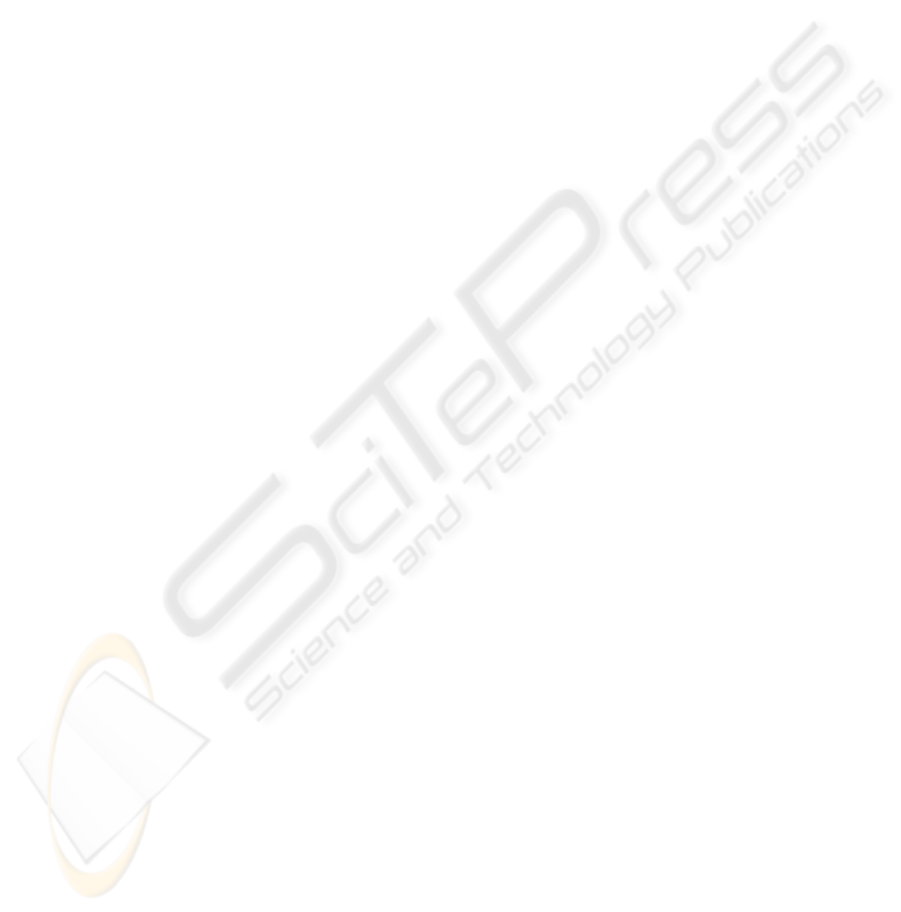JASTEG2000
Steganography for JPEG2000 Coded Images
Domenico Introna, Francescomaria Marino
DEE, Politecinico di Bari, via Re David 200, 70125 Bari, Italy
Keywords: Data hiding, steganography, privacy, JPEG2000.
Abstract: The steganography is the concept of making invisible a communication, and not only incomprehensible its
content (as cryptography does). This is generally achieved hiding a secret message into another one
(“cover”), which appears as the only object of the communication.
This paper proposes a steganographic method employing JPEG2000 images as “cover”. It reaches high
embedding even introducing a low distortion.
Experimental results have shown up to 35%-45% embedding rate, with 2 dB of distortion (in the worst case)
at 0.5 bpp and 30%-40% with less than 4 dB at 1.0 bpp. Comparing these results with those achieved by
JPEG2000-BPCS, it can be seen that our method produces considerably less post-embedding growth and
distortion (in some case, they differ for more than 5 dB).
1 INTRODUCTION
The word steganography comes from two Greek
words: στεγανοσ (i.e., steganos=hidden) and
γραϕια (i.e., graphia=writing). The join of these
words describes the concept of a communication
which hides not only its contents, but also its
existence. This is well different from cryptography,
which makes incomprehensible the meaning of a
communication, though this one can be seen by
everybody.
Steganographic techniques, usually exploit a
second perceptible message (“cover”), having
disjoined meaning by the secret message. The cover
works as a “Trojan horse”, being a container of the
secret message (F.A.P. Petitcolas, et al., 1999; S.
Katzenbeisser and F. A. P. Petitcolas, 2000).
The new technologies and, in special way, the
Internet and the information networks, require more
and more sophisticated strategies in order to prevent
the message privacy. Even different steganographic
techniques have been presented in literature, which
are suitable for many media and standard coding
involved with the Internet, at the best of our
knowledge, only two steganographic algorithms
dealing with the emerging JPEG2000 image
standard coding (JPEG2000 website; M. D. Adams,
2001) have been proposed: the algorithm proposed
by Pochi-Su and C.-C. Jay Kuo, 2003 and
JPEG2000-BPCS (Bit-plane Complexity
Segmentation) by H. Noda et al., 2002.
These two state-of-the-art methods follow
different philosophies. Briefly, the method proposed
by Pochi-Su and C.-C. Jay Kuo has the goal of
keeping constant the size of the cover image file, pre
and post the embedding. As a consequence, it is
necessary bounded for what concerns the embedding
capacity. Conversely, JPEG2000-BPCS aims at
getting high capacity, even with a low distortion,
though it gives less importance to the eventual post-
embedding growth.
In this paper, we consider the features of the
JPEG2000 standard coding, and how they can be
exploited in order to efficiently hide information.
We therefore define a technique for deciding which
bits of a given codeblock (and not all of them
without distinction) may be used for the embedding,
and how such embedding can be “reversibly
performed, i.e., allowing the receiver of correctly
recovering the hidden message.
We derive a flexible strategy which differentiates
the number of bits to be hidden into a coefficient
with respect to the absolute value of the same
coefficient, bounding (in a “someway relative”
sense) the introduced distortion. Its result is a high
embedding/low distortion steganographic method
which has been implemented in a software tool
329
Introna D. and Marino F. (2006).
JASTEG2000 - Steganography for JPEG2000 Coded Images.
In Proceedings of the International Conference on Security and Cryptography, pages 329-336
DOI: 10.5220/0002095403290336
c
SciTePress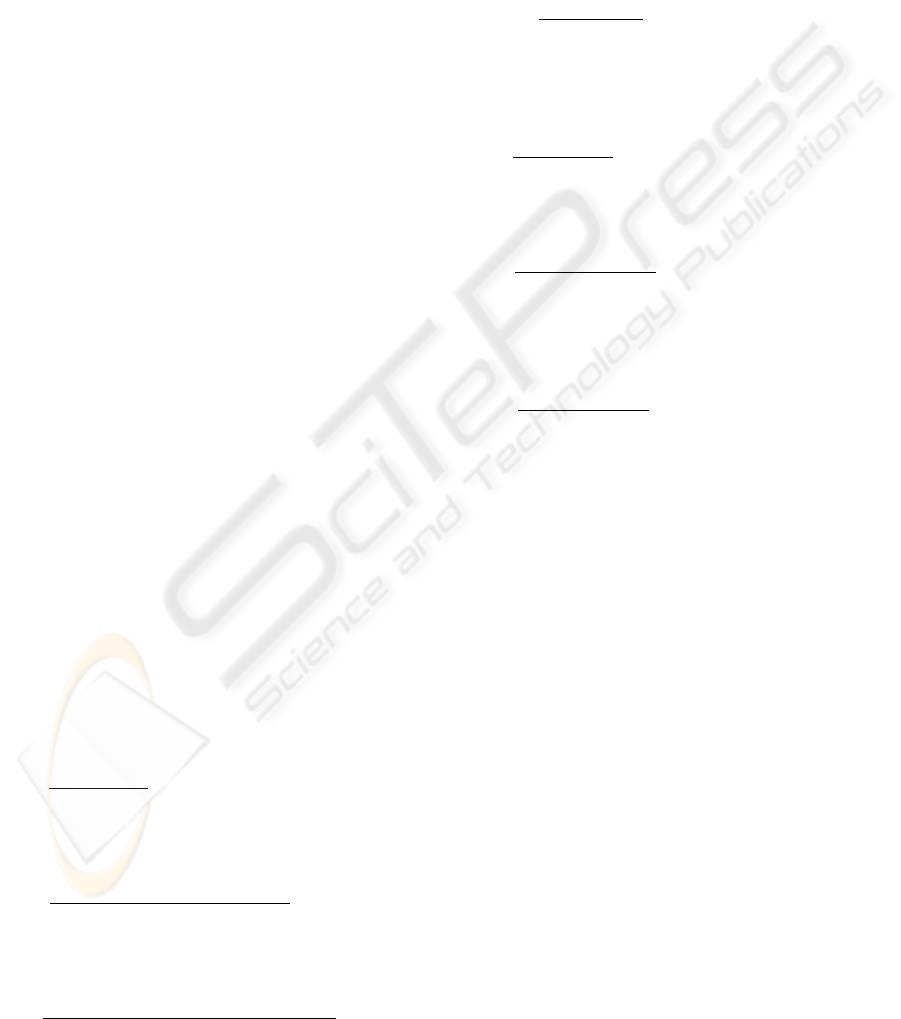(JASTEG2000 website) and experimentally
evaluated. In the worst cases, it gets up to 35%-45%
embedding rate with approximately 2 dB of
distortion, and up to 30%-40% embedding rate with
less than 4 dB of distortion, when applied on
benchmarks coded at 0.5 bpp and 1.0 bpp,
respectively. These results outperform those
achieved by other existing methods. In particular, we
will show that our method produces much less post-
embedding growth, getting a considerably lower
distortion, than JPEG2000-BPCS.
2 JPEG2000 STANDARD
JPEG2000 is a powerful coding technique for still
images, standardized as ISO 15444 in 2001. It
achieves impressive compression ratios even holding
a good image quality, overcoming the classical
JPEG. The key of this performance are the wavelets,
which constitute, in JPEG2000, the counterpart of
the Discrete Cosine Transform (DCT) in JPEG.
Wavelets (S. G. Mallat, 1989) use complex base
functions, with some coarse features akin to sine
waves. They also contain some detailed features like
pulse codes, thereby creating a set of fuzzy pixels
with variable-sized features, as opposed to DCT's
one-size-fits-all sine waves. Other interesting
(sometimes innovative) features of JPEG2000 are:
- it offers both lossless and lossy coding;
- it allows of modifying and coding any region of
the image, directly working on the compressed data
stream;
- it introduces the concept of Region of Interest
(ROI) of an image;
- it allows a flexible bitstream ordering;
- it has an improved error resilience of the
codestream;
- it provides a localized random access into an
image;
- it grants an efficient and accurate rate control.
More in detail, the processing steps of JPEG2000
are:
(a) DC-Shifting
: This step is applied on the
components having only positive values. It shifts the
range of these components from [0, 2
n
-1] to [-2
n-1
+1,
2
n-1
], n being the number of bits used for that
component.
(b) Multi-Component Transform
: This step is
needed in case of color images. It un-correlates the
color components either into YUV space (in case of
lossless coding) or into YCbCr space (in case of
lossy coding).
(c) Discrete Wavelet Transform (DWT)
: DWT is
the cornerstone of JPEG2000. The best way to
represent a signal using wavelets is to scan the entire
image for the “mother wave” that best represents
that particular image. However, this “mother wave”
would have to be attached to the image data, thereby
increasing the size of the compressed file. Instead,
of time, eliminating the need to send it along with
the file. These ones are LeGall 5/3 (for lossless
coding) and Daubechies 9/7 (for “lossy” coding).
(d) Quantization
: Scalar and uniform
quantization is applied on DWT coefficients. The
standard does not specify thresholds values, since
these ones can be decided by the user, basing on the
particular case. Anyway, the standard proposes a
method for determining them.
(e) ROI Scaling
: This is an optional functionality
in which, the wavelet coefficients related to regions
that the user has indicated as “relevant” are scaled
up. By this way, these Regions Of Interest (ROI)
gain quality during the next coding steps.
(f) EBCOT (Tier 1)
: In JPEG 2000, coding is
performed in two steps (tiers). In tier 1, the
quantized coefficients of each subband are
partitioned into codeblocks. These ones are
independently coded using an Embedded Bit-planes
Coding with Optimized Truncation (EBCOT).
(g) EBCOT (Tier2)
: Tier 2 optimally truncates
the bit-stream of each codeblock minimizing the
distortion due to the bit-rate constraint. Firstly,
candidate truncation points are selected in the
convex hull of the rate-distortion curve. Afterward,
when some codeblocks are collected, and a statistic
is available, the truncation point is selected among
these candidates in order to minimize the distortion.
In JPEG2000, this step produces information loss,
as well as quantization.
3 A STEGANOGRAPHIC
METHOD FOR JPEG2000
When steganography is applied to classical codecs
(e.g., JPEG), the embedding is generally cascaded to
the quantization, since this one is the main (and
often, the unique) lossy step. Nevertheless, this
approach is unsuitable for JPEG2000. In fact, in this
standard, the quantized coefficients can successively
be truncated in EBCOT Tier 2 in order to match a
particular bit rate, that the user could have required.
Therefore, steganography when applied to
JPEG2000 cover images needs a “down-coding/up-
decoding” scheme, as that one shown in Fig. 1. In it,
SECRYPT 2006 - INTERNATIONAL CONFERENCE ON SECURITY AND CRYPTOGRAPHY
330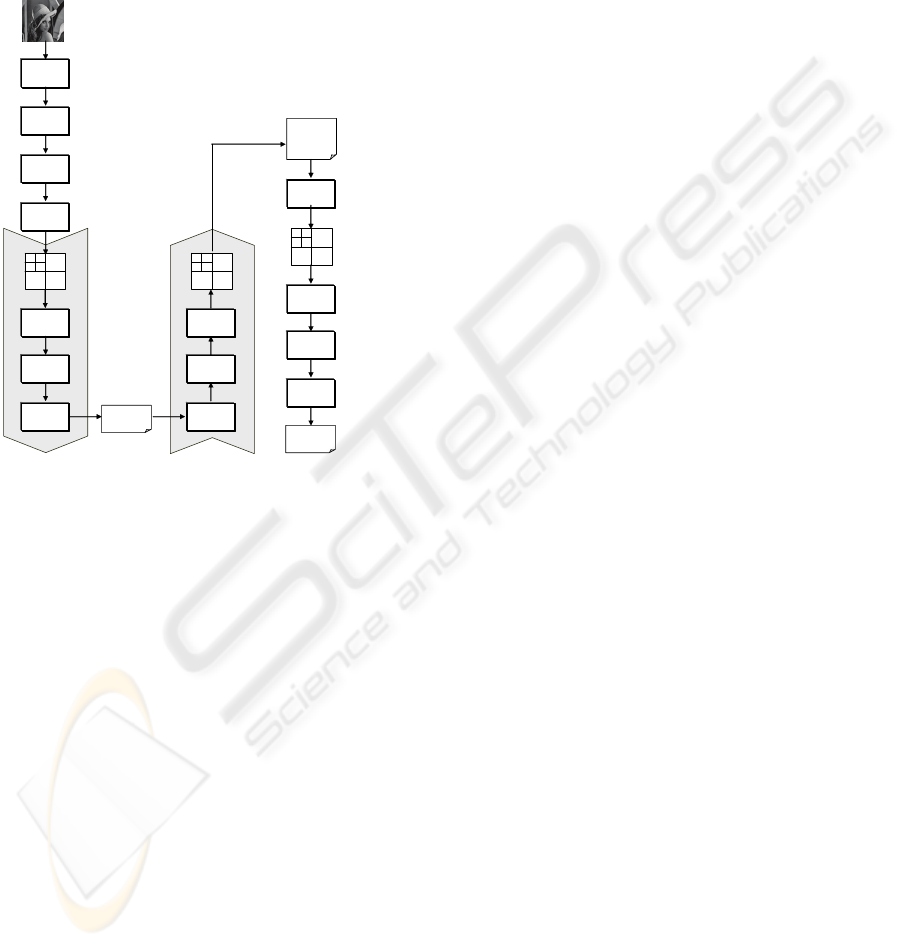and in the following, we will adopt the terms:
- CI, i.e., the Cover Image;
- SM, i.e., the Secret Message object of the
steganography;
- CICS, i.e., the Cover Image Code Stream (before
the embedding);
- MECS, i.e., the Message Embedding Code Stream
(after having embedded SM into CI).
COVER IMAGE
(CI)
”Hello
World!”
SECRET MESSAGE
(SM)
DC Shifting
MESSAGE-
EMBEDDING
CODE
STREAM (MECS)
(b)
MCT
(a)
DWT
(d)
Quantization
(c)
EBCOT Tier 1
(g)
EBCOT Tier 2
(f)
EBCOT Tier 1
EBCOT Tier 2
EBCOT Tier 1
(n)
EBCOT Tier 2
(without
rate-distortion)
(m)
(h)
(i)
Embedding
(k)
0010111
1100101
1010111
1101100
COVER IMAGE
CODE STREAM (CICS)
ROI Scaling
(e)
ROI Descaling
ROI Scaling
(l)
(j)
QUANTIZED &
TRUNCATED DWT
COEFFICIENTS
(
Matrix X
)
EMBEDDING
MESSAGE DWT
COEFFICIENTS
(Matrix X’)
QUANTIZED DWT
COEFFICIENTS
Figure 1: Steganographic approach to JPEG2000 cover
images.
Basically, a “cover image” is firstly coded in
JPEG2000 through steps (a)-(d), as described in
Section 2. After Quantization (d), for those cases
without bit-rate constraint (i.e., when the user has
not required any bit-rate, or when the final
achievable compression is not relevant), the
Embedding (k) can be directly performed, as
denoted by the dotted arrow, and the coding
completed through steps (l)-(n), taking care of do not
applying the rate distortion in step (n), since this
could truncate parts of the secret message.
Conversely, in case of bit-rate constraint, the
above mentioned “down-coding/up-decoding”
scheme is required. Steps (e)-(g) are carried out
(down-coding) in order to perform, in the EBCOT
Tier 2 (g), a bitplane truncation matching the
required bit-rate. These ones are then followed by
the up-decoding steps (h)-(j) which get newly back
the quantized and appositely truncated DWT
coefficients, to be used for the embedding in step
(k). Afterward, the final coding steps (l)-(n) are
performed, without applying now the rate distortion,
in step (n).
The embedding constitutes the soul of a
steganographic method. In particular, for JPEG2000,
this step should define: 1) how to select the bits to be
used as “hosts”, among those of the quantized and
truncated DWT coefficients, and 2) how to embed in
these host-bits the bits of the SM (making them
perfectly recoverable).
A fundamental feature of the proposed method is
constituted by its embedding technique. It not only
exploits the resolutions and the subbands which are
less sensible to the noise (as similar other
approaches do), but selects the number of secret bits
to be embedded into each coefficient, varying this
number on the base of the module of the related
coefficient: so doing, it “proportionally” corrupts
any coefficient. As a result, our method gets higher
PSNR than other steganographic methods, which
embed secret bits indifferently from the value of the
host-coefficients. Moreover, for what concerns the
strategy for selecting the host-bits, it has been
experimented in four different versions, providing
the user with four different compromises between
post-embedding growth and distortion. Because of
the space limitation, here only the "version 4" of the
method is described; details on the other versions of
the method can be retrieved on (JASTEG2000
website).
In the following, the above two issues are
discussed in detail.
3.1 Bits Embedding Technique
Let:
- α, a parameter input by the user and specifying the
maximum number of bits that might be potentially
modified in any DWT coefficient during the
embedding (obviously, higher α, higher the
embedding capacity and the introduced distortion);
- X, the matrix of the quantized and truncated DWT
coefficients x(i, j), constituting the input to the
Embedding step (k) in Fig. 1;
- PMS1 (|x(i, j)|), the Position of the Most
Significant “1” of |x(i, j)|, “0” being the position of
the LSB.
Therefore, for any non-zero coefficient x(i, j), we
can define the Position of the Most Significant
available Host Bit available for the embedding, as:
PMSHB(x(i,j)) = min{α-1, PMS1(|x(i,j)|-1)} (1)
In this scenario, the embedding in x(i, j) is
performed simply replacing the PMSHB(x(i, j))+1
least significant bits of x(i, j), with a same number of
bits of the secret message, getting a post-embedding
JASTEG2000 - Steganography for JPEG2000 Coded Images
331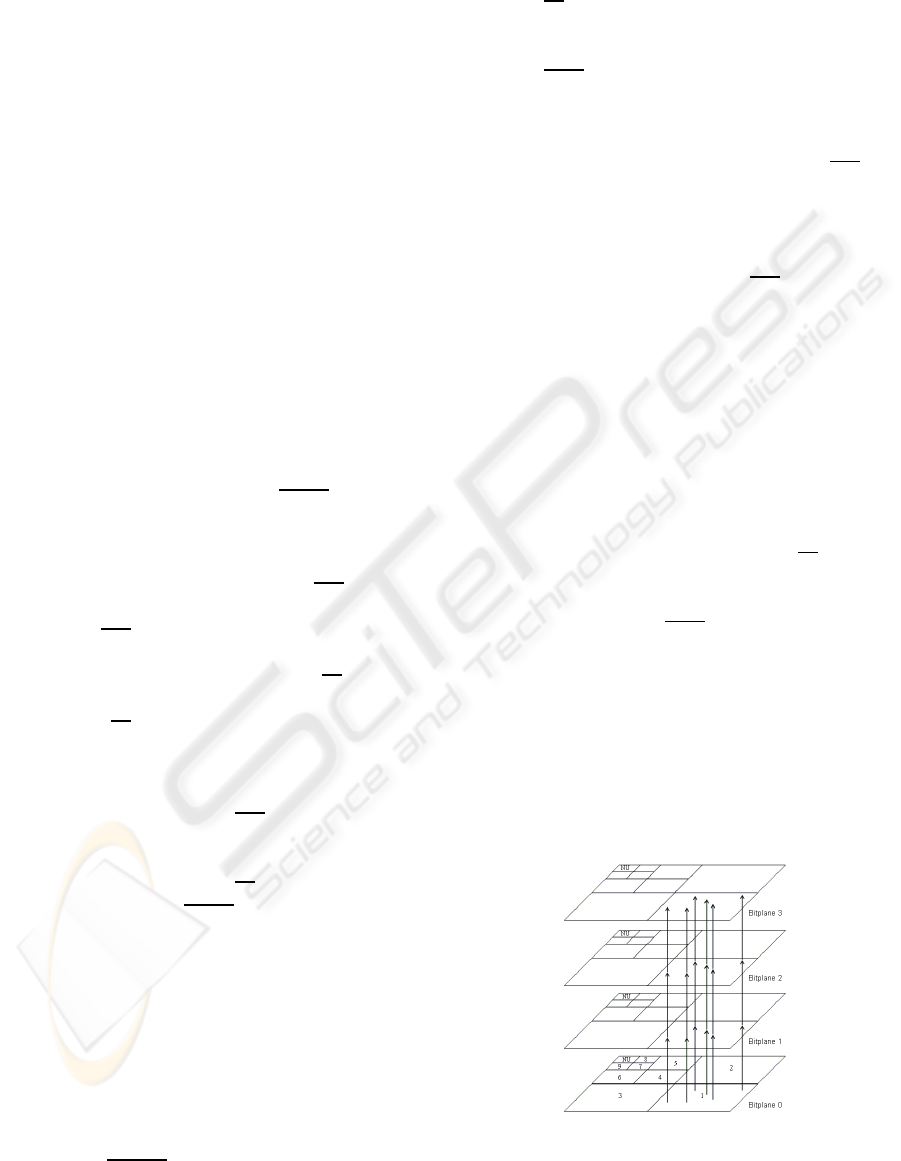value x'(i, j) to be coded (the coefficients {-1, 0,
1} having PMSHB(x)0 are not employed).
Note that since the embedding replaces the least
significant bits, the EBCOT Tier 2 in step (n) would
be performed without applying bit planes truncation,
as previously remarked. Because of this strategy, the
embedding modifies only the bits considered in the
refinement pass, leaving unaltered the other coding
passes. Moreover, because of (1), the number of bits
embeddable in x(i, j) –anyway, never more than α
grows with |x(i, j)|, bounding –in a “someway
relative” sense– the introduced distortion.
In the recovering step, the receiver, knowing α
and reading x'(i, j), can easily determine PMS1(|x'(i,
j)|) and PMSHB(x'(i, j)). Afterwards, supposed:
PMSHB(x'(i, j))=PMSHB(x(i, j)) (2)
(the case of PMSHB(x'(i, j))PMSHB(x(i, j)) is
discussed later) the receiver can simply reconstruct
the secret message, collecting the less significant
bits of any x'(i, j), up to the bit in position
PMSHB(x'(i, j)).
For the sake of clarity, examples of
embedding/recovering of secret bits 10010
into {-13,
4}, are provided in the following (α=4):
Embedding:
x=(-13)
10
=(11110011)
2-complement
; |x|=00001101;
PMS1(|x|)=3; PMSHB(x)=2 => 3 bits (100) can be
embedded up to the position 2; this generates:
x' =(11110001
)
2-complement
=(-15)
10
to be coded;
x=(4)
10
=(00000100)
2-complement
; |x|=00000100;
PMS1(|x|)=2; PMSHB(x)=1 => 2 bits (10
) can be
embedded up to the position 1; this generates:
x' =(00000101
)
2-complement
=(5)
10
to be coded;
Recovering:
x'=(11110001)
2-complement
=(-15)
10
; |x'|=00001111;
PMS1(|x'|)=3; PMSHB(x')=2 => 3 bits can be
recovered up to the position 2: 100
x'=(00000101)
2-complement
=(5)
10
; |x'|=00000101;
PMS1(|x'|)=2; PMSHB(x')=1; => 2 bits can be
recovered up to the position 1: 10
Recovered message: 10010
Nevertheless, even (2) holds for positive values
of x(i, j), particular sequences of embedded bits (i.e.,
either a sequence of all '0' or a sequence of all '1'),
might modify negative values of x(i, j) into values
x'(i, j) causing:
PMSHB(x'(i, j)) PMSHB(x(i, j)) (3)
which is the negation of (2).
Obviously, these values, if effectively coded,
will generate an incorrect message recovering. This
is better evidenced by the following examples where
the secret bits 001111
are embedded into {-6, -16},
causing an incorrect recovering:
x=(-6)
10
=(11111010)
2-complement
; |x|=00000110;
PMS1(|x|)=2; PMSHB(x)=1; after having
embedded 00
: x' =(11111000)
2-complement
=(-8)
10
;
x=(-16)
10
=(11110000)
2-complement
; |x|=00010000;
PMS1(|x|)=4; PMSHB(x)=3; after having
embedded 1111
: x' =(11111111)
2-complement
=(-1)
10
;
Recovering (in this case it will result incorrect):
x'=(11111000)
2-complement
=(-8)
10
; |x'|=00001000;
PMS1(|x'|)=3; PMSHB(x')=2; => 3 bits are
supposed to be recovered up to the position 2: 000
x'=(11111111)
2-complement
=(-1)
10
; |x'|=00000001;
PMS1(|x'|)=0; PMSHB(x')=-1; => 0 bits are
supposed to be recovered, i.e., this coefficient
appears to be unused during the embedding.
Erroneously recovered message: 000
Therefore, in all the cases verifying (3), a post-
processing of x'(i, j) is needed in order to assure a
correct message recovering. This is made
complementing the bit of x'(i, j) in position
PMSHB(x(i, j))+1, by this way, getting a new value
verifying (2). It is worth to note that this correction
is carried out during the embedding, by the
“sender”, which well knows PMSHB(x). For the
sake of clarity, the examples previously considered
became (after being post-processed):
x'=(11111000)
2-complement
; after complementing
the bit in position 2: x'=(11111100
) with
PMSHB(x')=1;
x'=(11111111)
2-complement
after complementing the
bit in position 4: x'=(11101111
) with PMSHB(x')=3;
As a proof of the effectiveness of the introduced
corrections, it can be seen that now PMSHB(x')
PMSHB(x), that will allow a perfect recovering.
3.2 Bits Selection Strategy
The "version 4" of our method selects the
coefficients x(i, j) starting from the HH orientation at
the highest resolution, and continues as denoted by
the order shown in Fig. 2.
Figure 2: “Vertical” bits embedding (decomposition in 3
levels of DWT with 4 bitplanes. NU=Not Used).
SECRYPT 2006 - INTERNATIONAL CONFERENCE ON SECURITY AND CRYPTOGRAPHY
332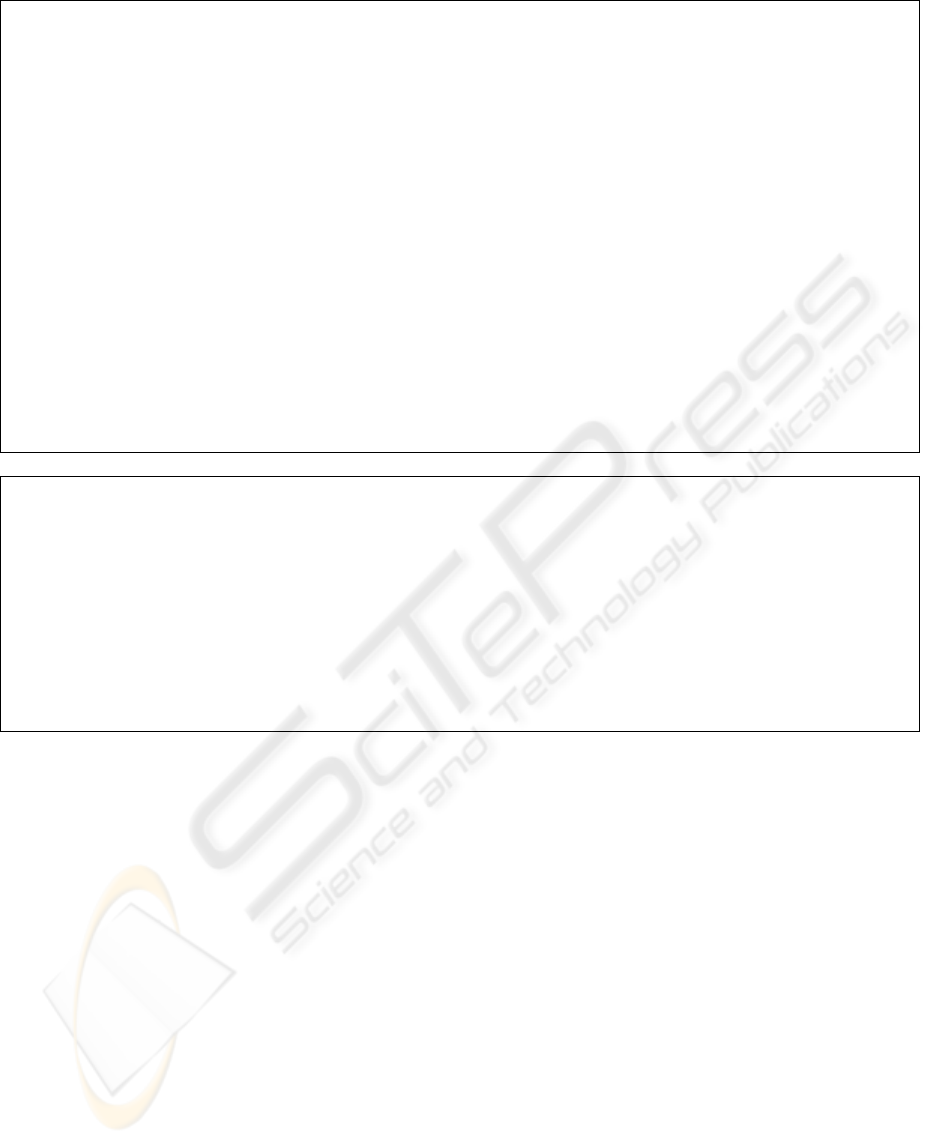0. From the CI, build X, the matrix of coefficients, through the steps (a)-(j) of
Fig. 1.
1. create and initialize a second matrix X' = X;
2. while (there are secret bits to be embedded) AND (host-bits are available) do
3. select a subband S according to the given order (Fig. 2);
4. consider the next coefficient x(i, j) into subband S, according to a given
scanning path;
5. given α, evaluate PMS1(|x(i, j)|) and PMSHB(x(i, j));
6. modify x’(i, j) by replacing its PMSHB(x(i, j))+1 less significant bits
with secret bits;
7. discard the just embedded PMSHB(x(i, j))+1 bits of the secret message;
8. if PMSHB(x'(i, j)) PMSHB(x(i, j))
9. correct x’(i, j) by complementing its bit in position PMSHB(x(i, j))+1;
10. end while;
11. if (there are secret bits to be yet embedded) do
12. output “SECRET MESSAGE TOO LONG – EMBEDDING NOT COMPLETED
RETRY INCREASING EITHER α OR THE BIT RATE OF THE COVER IMAGE”
13. exit;
14. end if;
15. apply steps (l)-(n) of Fig. 1 on X’ without bit planes truncation;
16. end.
(a)
0. From the MECS, obtain X' performing the reverse EBCOT Tier2, reverse EBCOT
Tier1 and ROI Descaling;
1. while there are secret bits to be recovered do
2. select a subband S according to the given order (Fig. 2);
3. consider the next coefficient x(i, j) into subband S, according to a given
scanning path;
4. given α, evaluate PMS1(|x'(i, j)|) and PMSHB(x'(i, j));
5. collect the PMSHB(x'(i, j))+1 less significant bits of x'(i, j) into the
recovered message;
6. end while;
7. output the recovered message;
8. end.
(b)
Figure 3: Pseudo code. Message embedding (a); message recovering (b).
As soon as a coefficient x(i, j) is considered, all
its PMSHB(x(i,j))+1 least significant bits are
replaced in a “vertical” fashion (i.e., along any bit
plane) by a same number of secret bits, as shown by
the arrows, starting from the least significant one.
Pseudo code of this version of our
steganographic method is given in Fig. 3. In Fig. 3.a,
lines 2-10 perform the embedding. The subband S in
line 3 is selected according to the order shown in
Fig. 2. The coefficient x(i,j) is selected into S in line
4 according to any pre-established scanning path
(raster or what ever); an additional possibility might
be that one of discarding the pixels belonging to
region of interest in order to do not deteriorate them
during the embedding. Lines 11-13 terminate the
procedure, sending a warning, in case the embedding
cannot be completed because the available host-bits
are less than the secret bits to be embedded.
4 EXPERIMENTAL RESULTS
In order to evaluate HELD, we have implemented
the software tool “JASTEG2000”. JASTEG2000 is a
visual environment whose name is two-fold: 1) it is
a tribute to the well known freeware JASPER
(Jasper website), that we have used for developing
the codec; 2) it recalls its main goal:
STEGanography for JPEG2000 cover images”.
We have experimentally conducted some studies,
in order to examine the post-embedding growth rate
and distortion, using as benchmarks three well-
known 512x512 pixels images (“Barbara”, “Lena”
and “Mandrill”). “Barbara” and “Lena” are grey-
scale images at 8bpp; “Mandrill” is a true-color
image at 24bpp.
These tests are described in paragraphs 4.1 and
4.2, respectively. Afterward, a comparative analysis,
using as terms of comparison the other
JASTEG2000 - Steganography for JPEG2000 Coded Images
333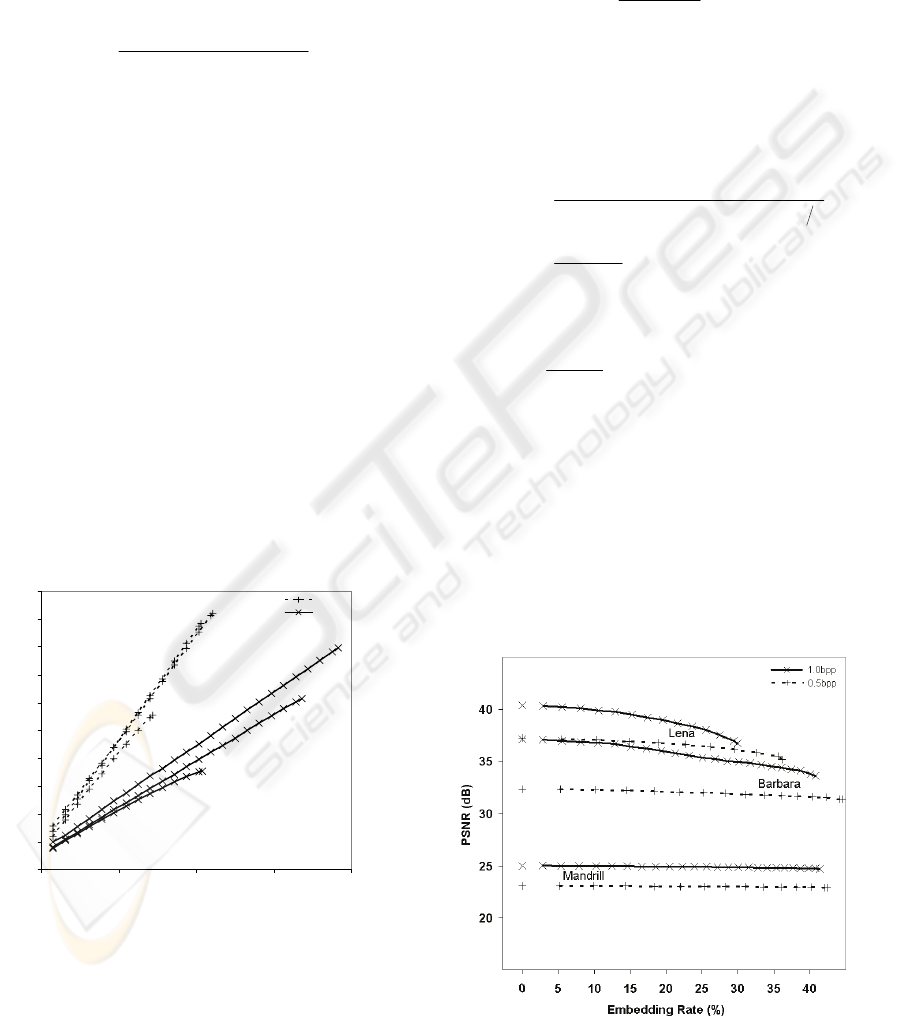steganographic approaches to JPEG2000 available in
literature is reported.
4.1 Growth Rate
A first experiment evaluates the impact of the
embedding in terms of growth of MECS, measuring
the Growth Rate, defined as:
%100
size CICS
size CICS-size MECS
=RateGrowth
(4)
We randomly generated a sequence of bits
(constituting the SM) and performed different tests,
increasing, test after test, the amount of information
to be hidden. Though the optimal value of α (i.e., the
maximum number of host-bits per coefficient)
should be tentatively found for each cover image
and at any bit rate, we reduced the degrees of
freedom, using α=4 for the entire set of tests, after
having verified that this choice represented a good
compromise between embedding capacity and
introduced distortion.
Fig. 4 shows the results of this experiment. It
reports the “Growth Rate vs Embedded kbits”
characteristics obtained by steganography performed
onto the test images coded both at 0.5 and at 1.0
bpp, with 6 DWT resolutions, and after having
applied the component transform on the colour
image “Mandrill”.
It is evident that the shown characteristic well
fits a linear trend which makes our method
particularly attractive, since the effect of the growth
rate can be easily predicted.
0
10
20
30
40
50
60
70
80
90
100
0 50 100 150 200
Embedded Message (kbit)
Growth Rate (%)
0.5bpp
1.0bpp
Lena
Barbara
Mandrill
Lena
Barbara
Mandrill
Figure 4: Growth Rate vs Embedded Message for
Mandrill, Barbara and Lena coded at 0.5 and 1.0 bpp.
4.2 Post-Embedding Distortion
A second experiment focused on the distortion
introduced by the steganography onto the cover
images. In order to produce measures comparable
with those reported by their authors for JPEG2000-
BPCS, we have considered the “PSNR vs the
Embedding Rate” characteristic.
In this context, the Embedding Rate evaluates the
integration of SM inside CI, and it is defined as:
%100
sizeCICS
sizeSM
=RateEmbedding
(5)
while PSNR measures the quality loss of the
message-embedding image with respect to the cover
image (i.e., the image itself, before the embedding),
and it is defined (for colour and grey scale,
respectively) as:
()
++
=
=
)(
255
log10
3)()()(
255
log10
2
10
2
10
YMSE
BMSEGMSERMSE
PSNR
(6)
with:
()
∑∑
==
×
=
M
i
N
j
cjiPcjiP
NM
cMSE
11
2
),,(),,('
1
)(
(7)
where MxN is the size of the image, in pixels; and
P(i, j, c) and P'(i, j, c) are the numerical values of the
pixel (i, j) for the component c, before and after the
embedding, respectively.
This experiment was conducted measuring
PSNR and Embedding Rate in each one of the
incremental test conducted in the previous
experiment. The obtained results are shown in Fig.
5, where the points with embedding rate = 0 denote
the quality loss due to the only compression.
Figure 5: PSNR vs Embedding Rate for Mandrill, Barbara
and Lena coded at 0.5 and 1.0 bpp.
SECRYPT 2006 - INTERNATIONAL CONFERENCE ON SECURITY AND CRYPTOGRAPHY
334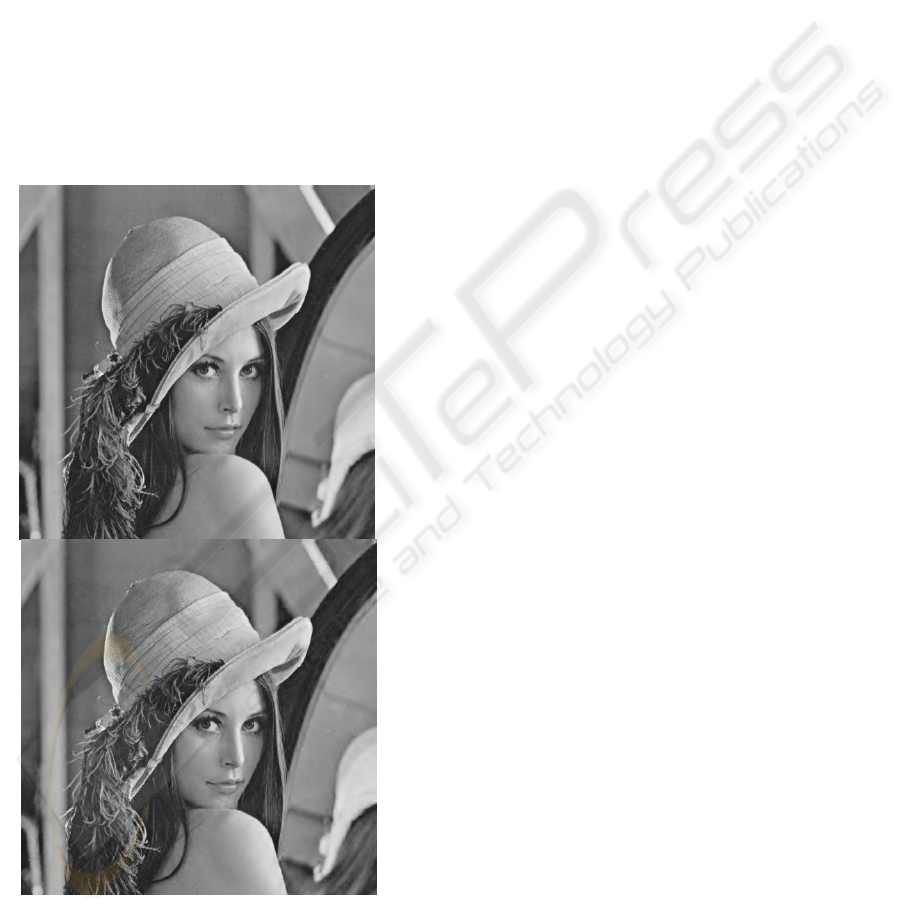We can observe that the PSNR, though slightly
decreasing at higher embedding rates, remains
almost constant in Mandrill, since it varies (in the
considered interval of embedding rate: 0%-45%) in a
range of only 0.16 dB and of 0.31 dB, for the coding
at 0.5 bpp and 1.0 bpp, respectively. Conversely, a
more perceptible decrement in the PSNR is visible
for the images Barbara coded at 0.5 bpp (1 dB:
from 32.34 dB at 0% down to 31.37 dB at 45%), and
more pronouncedly for Lena coded at 0.5 bpp (2
dB: from 37.23 dB at 0% down to 35.15 dB at 36%).
Instead, the curves related to Barbara and Lena
(coded at 1.0 bpp) decrease according to a slightly
convex and almost linear trend.
Concluding, in order to provide an evaluation
even from a perceptive point of view, we show in
Fig. 6 the test image Lena (the worst benchmark, in
the sense of PSNR) in case of an embedding rate
Figure 6: Steganography onto Lena coded at 1.0 bpp
(Embedding rate=30%). pre (top) and post the embedding
(bottom).
of 30% (coding at 1.0 bpp). It can be seen that the
introduced distortion is not perceptible with respect
to the quality loss already caused by the
compression.
4.3 Comparative Analysis
Only two other steganographic algorithms have
already been proposed for JPEG2000: JPEG2000-
BPCS (H. Noda et al. 2002) and the method
proposed by Po-Chyi Su and C.-C.J. Kuo, 2003.
It is worth to note that method by Po-Chyi Su
and C.-C.J. Kuo follows a philosophy completely
different from our method. In fact, its main goal
consists of holding constant the size of the code
stream after the embedding (size of MECS = size of
CICS). Consequently, it allows good embedding
capacity only at high bit-rates. Due to these different
features, in this comparative analysis it is considered
only for the sake of completeness.
Conversely, JPEG2000-BPCS, rather than
holding constant the size of the code stream, aims at
preserving a high PSNR, even getting a high
embedding capacity. Its goal is the same of the
algorithm proposed in this paper. Therefore, the
comparison of our method vs JPEG2000-BPCS is
more significant.
1) growth rate: The only available data for what
concerns the growth rate reached by JPEG2000-
BPCS are 29.8% and 32.8% caused when 2412
bytes and 7332 bytes are embedded into Barbara in
case of coding at 0.5bpp and at 1.0bpp, respectively.
These ones are well higher than those of our method
(22.4% and 24.9%) at the same conditions.
As previously said, no growth (for any allowed
dimension of the embedded message) is generated
by the method by Po-Chyi Su and C.-C.J. Kuo, since
it has been conceived appositely aiming at this goal.
2) post-embedding distortion: In Fig. 7 the
characteristics of the steganographic algorithm
proposed by Po-Chyi Su and C.-C.J. Kuo are
reported. They have been obtained thanks to Dr. Po-
Chyi Su, that has kindly provided the software
implementing his method. As already said, this
method does not allow high embedding rate at 1.0
bpp (only 6.8% for Lena and 3.4% for Barbara).
Moreover, it decreases the PSNR, much faster than
JPEG2000-BPCS and our method do. It pays this
price in order to achieve a post-embedding “growth
0”.
A more significant comparison can be performed
with respect to JPEG2000-BPCS. In Fig. 8 we
propose the “PSNR vs Embedding Rate” diagram,
related to the performance of JPEG2000-BPCS, as it
was reported by their authors. Comparing this figure
JASTEG2000 - Steganography for JPEG2000 Coded Images
335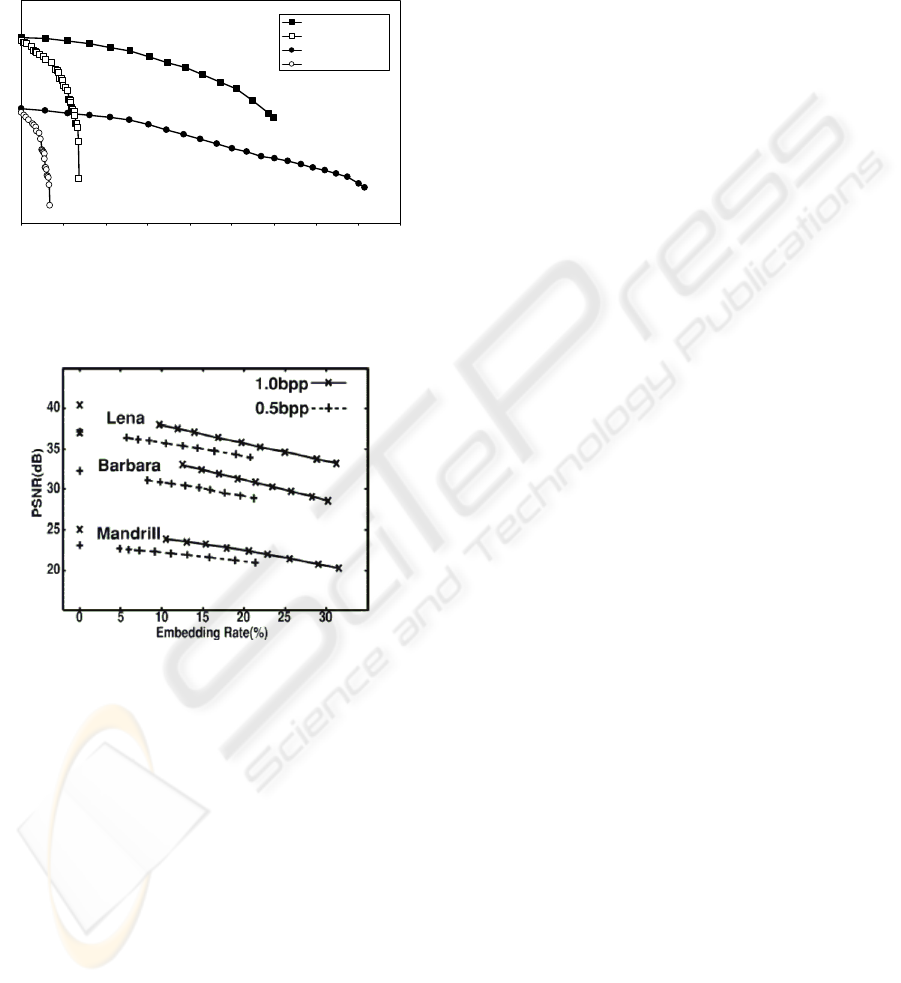with the Fig. 5, it can be seen that, for any test
image, our method performs significantly better than
JPEG2000-BPCS, inducing much lower distortion
(in some case, more than 5 dB), and getting higher
embedding rates than those reported in Fig. 8 for
what concerns the images coded at 0.5 bpp.
32
34
36
38
40
42
0 5 10 15 20 25 30 35 40 45
Em bedding Rate [%]
PSNR [dB]
Lena - Our Method
Lena - Po Chyi Su
Barbara - Our Method
Barbara - Po Chyi Su
Figure 7: PSNR vs Embedding Rate in case of
steganography by our method and by Po-Chyi Su and C.-
C.J. Kuo Algorithm, performed onto Lena and Barbara
coded at 1.0 bpp.
Figure 8: PSNR vs Embedding Rate in case of
steganography by JPEG2000-BPCS, as shown in (H. Noda
et al., 2002).
5 CONCLUSION
A new steganographic algorithm, suited for
JPEG2000 cover images, has been introduced. It is
based on an embedding technique that, not only
corrupts the resolutions that are less sensitive to the
noise, but also determines the number of bits to be
embedded into a single DWT coefficient, basing on
its magnitude.
This particular feature results in a low “relative”
distortion. Its performances reachable by these four
versions have been experimentally analyzed using
well known benchmarks, and the post-embedding
growth and distortion measured.
In particular, up to 35%-45% embedding rate
with approximately 2 dB of distortion in the worst
cases (for the benchmarks coded at 0.5 bpp), and up
to 30%-40% embedding rate with less than 4 dB of
distortion in the worst cases (for coding at 1.0 bpp)
have resulted. Moreover, for what concerns the
growth of the codestream, it gets a very interesting
feature: it performs linearly, making the effect of
this phenomenon easily predictable.
The proposed method has been compared also
with other existing steganographic methods suited
for the JPEG2000 standard, in particular with
JPEG2000-BPCS, which similarly to our method,
aims at getting high embedding, introducing low
distortion. The comparison has put in result that our
method:
- produces considerably less growth;
- gets higher embedding rates at low bit rate coding;
- induces lower distortion (in some case, it differs
from JPEG2000-BPCS for more than 5 dB).
ACKNOWLEDGEMENTS
The authors would thank Dr. Pochyi-Su (National
Central University, Taiwan) for having kindly
provided the source code of his steganographic
method.
REFERENCES
F.A.P. Petitcolas, R.J. Anderson and M.G. Kuhn, 1999.
Information Hiding – A Survey. Proceedings of the
IEEE, vol.87, no.7, pp. 1062-1078.
S. Katzenbeisser and F. A. P. Petitcolas, 2000. Information
Hiding Techniques for Steganography and Digital
Watermarking. Norwood, MA: Artech House.
JPEG2000 website: http://www.jpeg.org/JPEG2000.html
M. D. Adams, 2001. The JPEG-2000 still image
compression standard. ISO/IEC JTC 1/SC 29/WG 1,
Tech. Rep.
Po-Chyi Su and C.-C.J. Kuo, 2003. Steganography in
JPEG2000 compressed images. IEEE Transactions on
Consumer Electronics, V. 49, N. 4, p. 824-832.
H. Noda, J. Spaulding, M.N. Shirazi and E. Kawaguchi,
2002. Application of bit-plane decomposition
steganography to JPEG2000 encoded images. IEEE
Signal Processing Letters, V. 9, N. 12, pp. 410-413.
JASTEG2000 website: http://dee.poliba.it/dee-web/
marinoweb/JASTEG2000.html
S. G. Mallat, 1989. A theory for multiresolution signal
decomposition: The wavelet representation. IEEE
Trans. Pattern Anal. Machine Intell., vol. 2, Dec., pp.
674–693.